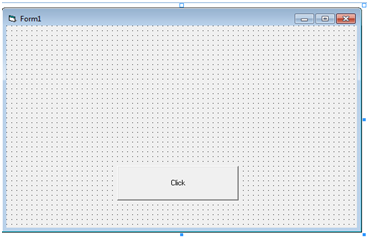Pages

vb_2014_slip 7SLIP 7_1

Option Explicit

Private Sub cmdFebo_Click()
Dim number As Integer
number = Val(InputBox("Enter a Number"))
Dim prev_num As Integer
Dim cur_num As Integer
Dim next_num As Integer
Dim i As Integer
prev_num = 0
cur_num = 1
Print cur_num
For i = 1 To number
next_num = cur_num + prev_num
Print next_num
prev_num = cur_num
cur_num = next_num
Next
End Sub